Next: Free Streamline Theory Up: Two-Dimensional Potential Flow Previous: Conformal Maps

# Schwarz-Christoffel Theorem

The Schwarz-Christoffel theorem is an important mathematical result that allows a polygonal boundary in the-plane to be mapped conformally onto the real axis,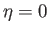, in the-plane. It is conventional to map the region inside the polygon in the-plane onto the upper half,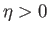, of the-plane. If the interior angles of the polygon are,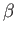,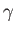,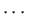then the appropriate map is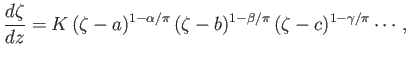(6.79)

where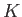is a constant, and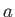,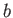,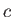,are the (real) values ofthat correspond to the vertices of the polygon (Milne-Thompson 2011).

It is often convenient to take the point in the-plane that corresponds to one of the vertices of the polygon--say, that given by--to be at infinity. In this case, the factor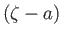in Equation (6.79) becomes effectively constant, and can be absorbed into a new constant of proportionality,.Consider, for example, a semi-infinite strip in the-plane, for which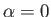,, and. This is mapped onto the upper half of the-plane, with the zero-angle vertex corresponding to a point at infinity in the-plane, by means of the transformation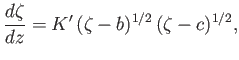(6.80)

whereis real and positive. We can integrate the previous expression to give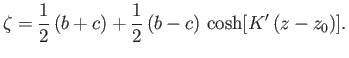(6.81)

Here, the pointsand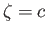in the-plane correspond to the vertices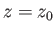and, respectively, in the-plane. (See Figure 6.12.)

Suppose that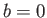,,, and. In this case, the transformation (6.81) becomes(6.82)

which implies that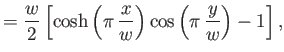(6.83)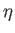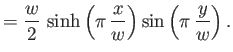(6.84)

The transformation (6.82) maps the semi-infinite strip in the-plane that is bounded by the lines,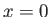, andinto the upper half of the-plane. The transformation also maps the origin of the-plane to the origin of the-plane, and the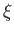-axis to the lines,, and. Now, in the-plane,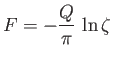(6.85)

is the complex potential associated with a line source of strength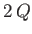, located at the origin. By symmetry, the-axis corresponds to a streamline, and can, therefore, be replaced by a rigid boundary. It follows that, in the-plane, the same potential is that due to a line source, of strength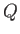, located in the lower left-hand corner of a semi-infinite strip bounded by rigid planes at,, and. (Incidentally, the source strength in the-plane is, rather than, because, by symmetry, half the output from the source in the-plane goes below the-axis and, therefore, does not map to the semi-infinite strip in the-plane.) The corresponding stream function is(6.86)

and is illustrated in Figure 6.13.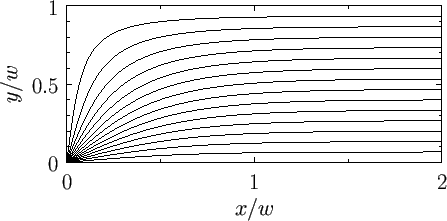An infinite strip in the-plane is a polygon with two zero-angle vertices, both at infinity. The required transformation follows from Equation (6.79) by setting,, and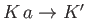. Thus, we obtain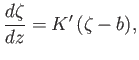(6.87)

which can be integrated to give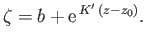(6.88)

This transformation maps the pointto the point, the-axis to the two lines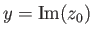and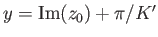, and the upper half of the-plane to the region between the linesand, as illustrated in Figure 6.14. It is clear that the transformation (6.60), studied in the preceding section, is just a special case of the transformation (6.88).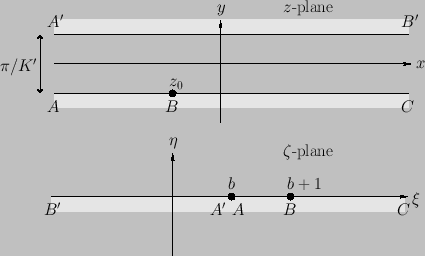Next: Free Streamline Theory Up: Two-Dimensional Potential Flow Previous: Conformal Maps
Richard Fitzpatrick 2016-03-31